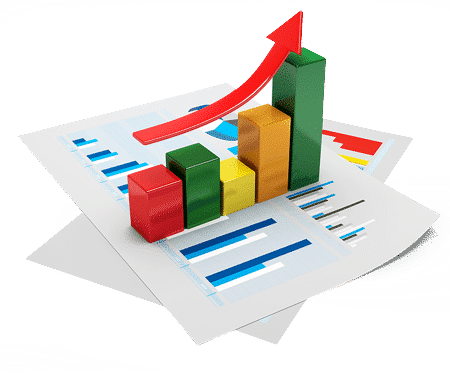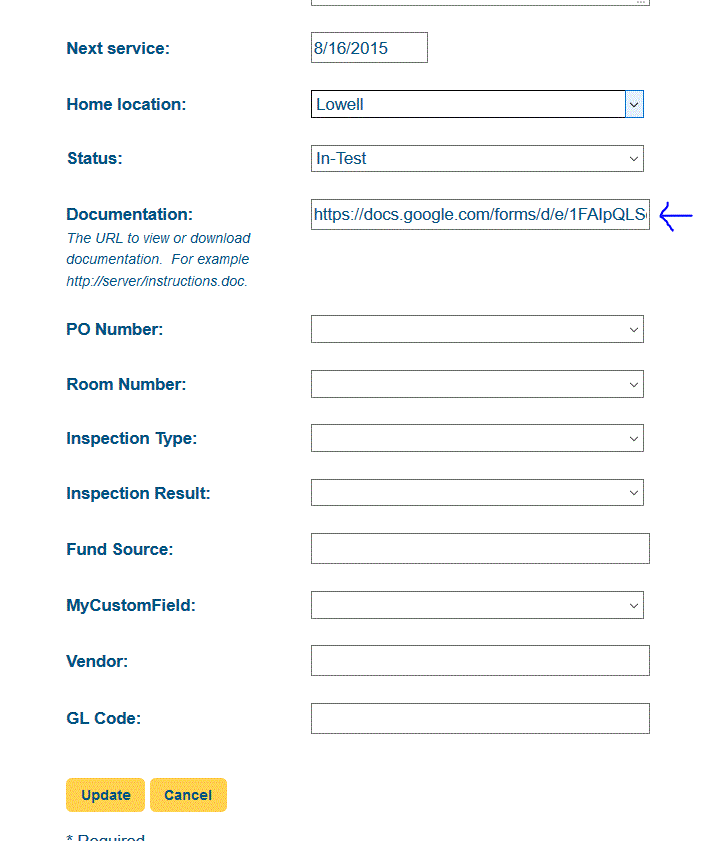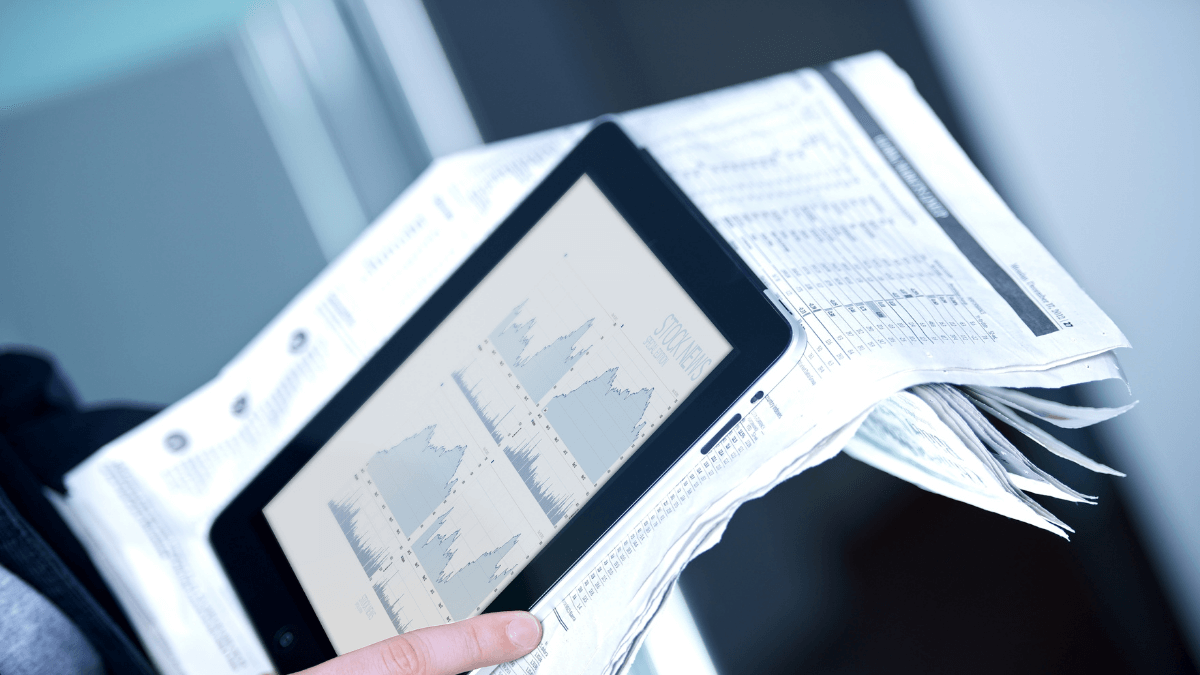1.800.513.5778

BOOK A CONSULTATION
HELP

# Appreciating Depreciation – Choosing the Best Software to Calculate Depreciation

3 min

In order to choose the right software for calculating depreciation, you first need to understand what depreciation is, how it can affect your business, and the different methods out there for calculating it. All in all, it’s pretty simple to understand. Once you do, choosing software to help you calculate and track depreciation is easy.## What Is Depreciation?

According to Merriam-Webster, the definition of depreciate is “to deduct from taxable income a portion of the original cost of a business asset over several years as the value of the asset decreases,” or “to lower the price or estimated value of (an asset).” Although this may seem complicated at first, depreciation is simply the amount the value of a particular asset drops over time due to age, wear and tear, or a lack in value. Every asset in your business – from your computer to your vehicles, and even to the machinery used on a production line – depreciates over time. Calculating and tracking depreciation helps you better understand your business’s worth.

## Methods for Calculating Depreciation

There are two main methods that businesses use to calculate the depreciation of assets. These are the straight-line method and the accelerated depreciation method.

• Straight-Line Depreciation – This is by far the most commonly-used method of depreciation. It takes the acquisition or purchase price of a single asset, subtracts its salvage value, and then divides it by the asset’s total estimated life in years. For example, if you purchase a car for \$50,000, it’s salvage value is \$500, and it has an expected lifespan of five years, you would subtract \$500 from \$50,000 to get \$49,500, and then divide that amount by five for an annual depreciation rate of \$9,900 per year. The depreciation rate is constant, so it is easier for you to make predictions about the future.
• Accelerated Depreciation Method – This method provides a significant tax benefit over the straight-line depreciation method since it allows for larger write-offs in earlier years and smaller write-offs later down the road. It is generally calculated using the DDB method, which stands for Double Declining Balance. To put it as simple as possible, the depreciation rate follows the equation 2/x * (total acquisition cost – accumulated depreciation) in the first year, and 2/x * (asset’s value on the balance sheet) for years two through five, where x is the asset’s estimated life in years.

Thus, the first year depreciation would be \$20,000, which would allow your company to report less income for that year and therefore pay fewer taxes. Although the depreciation rate is lower for the following four years, the reduction in taxes helps to offset some of the cost associated with the asset’s initial purchase.

## Choosing the Right Software

Now that you understand what depreciation really is and the two main methods of calculating it, choosing a software to help you calculate and track depreciation is much, much simpler. For the most part, you’ll want to find software that is capable of tracking multiple assets, providing you with instant, real-time reports for the purposes of estimating your taxes, and integrating with your favorite bookkeeping software to keep things simple all around. Doing it on your own can be time-consuming, and there’s always a margin for human error. Software keeps things simple and handles everything behind the scenes based on the depreciation method you choose.

With all of the software out there that is capable of calculating and tracking the depreciation of your assets, it makes sense to choose one that works with barcodes or QR labels. This way, you have access to information for each individual asset at a glance, all without having to dig through huge spreadsheets or piles of paperwork.

Using GoCodes to Calculate Fixed-Asset Deprecation

Similar Posts